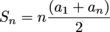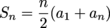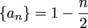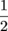## Arithmetic Series

Time to crank it up another notch with arithmetic series. The only difference between an arithmetic series and an arithmetic sequence is that the series is just the sum of all the terms.

There is, however, one piece of information we here at Shmoop feel we must bestow upon you. We're talkin' the formula for the sum of an arithmetic series. The good news here is that you just need to remember a formula instead of adding up a bunch of terms like before. The bad news is you need to remember a formula. Here it is:Sn is the notation typically used for a partial sum. A partial sum is just the sum of the first n terms. If the sum of the first 5 terms was 72, you could write S= 72.

Even though regular old textbooks might write this formula as, we actually like our version better. This allows us to remember the sum of an arithmetic series as the number of terms times the average of the first and last term.

### Sample Problem

Find the 8th partial sum of the sequence {an} = -10 + 4(n – 1).

Translation: "8th partial sum" just means "the sum of the first 8 terms."

This problem is gift-wrapped nicely for us since the rule is already in the explicit form of an arithmetic sequence:

{an} = a1 + d(n – 1)

We just need to find n, which is 8, a1, which is -10, and a8, which is -10 + 4(8 – 1) = 18. These values are then plugged into our partial sum formula and simplified to find the solution.Not convinced? We can take the long way around and add up the first 8 terms too…

-10 – 6 – 2 + 2 + 6 + 10 + 14 + 18 = 32

### Sample Problem

Find the 22nd partial sum of.

This problem is a little more challenging since we don't have a sequence in our typical arithmetic form. That means we need to first make sure this is arithmetic, then develop a rule that works. Let's check out a few terms.So we definitely have an arithmetic-type flow here. The common difference is –and the first term is.

Next, to find our partial sum, we need the last term. In this case, it's the 22nd term. We can use the given rule to find it.Finally, we can use our partial sum formula to find our solution.Next.

### Sample Problem

Find the sum of 74 + 66 + 58 + … + 10 + 2.

Once again, we need to know three things to find the sum: the number of terms, the first term, and the last term. In this case, the first and last terms are pretty easy to locate. The issue comes in knowing how many terms exist. To do this we can put together a rule and then solve.

Remember, the general form for an arithmetic sequences is {an} = a1 + d(n – 1). So the rule in this case will {an} = 74 – 8(n – 1) since a1 = 74 and d = -8.

Next, we must find the number, n, in the series that the term 2 corresponds with. To do this, we can set 2 equal to our formula and solve for n.

2 = 74 – 8(n – 1)
2 = 74 – 8n + 8
2 = 82 – 8n
8n = 80
n = 10

This means that 2 is the 10th term. We have 10 terms in our series that starts at 74 and finishes at 2. This is fantastic news because we can now finally find the 10th partial sum, S10.Done and done.